Counting Process Style of Input

In the counting process formulation, data for each subject are identified by a tripleof counting, at-risk, and covariate processes. Here,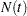indicates the number of events that the subject experiences over the time interval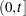;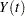indicates whether the subject is at risk at time(one if at risk and zero otherwise); and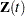is a vector of explanatory variables for the subject at time. The sample path of
is a step function with jumps of size +1 at the event times, and. Unlesschanges continuously with time, the data for each subject can be represented by multiple observations, each identifying a semiclosed time interval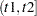, the values of the explanatory variables over that interval, and the event status at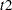. The subject remains at risk during the interval, and an event might occur at. Values of the explanatory variables for the subject remain unchanged in the interval. This style of data input was originated by Therneau (1994).

For example, a patient has a tumor recurrence at weeks 3, 10, and 15 and is followed up to week 23. The explanatory variables are Trt (treatment), Z1 (initial tumor number), and Z2 (initial tumor size), and, for this patient, the values of Trt, Z1, and Z2 are (1,1,3). The data for this patient are represented by the following four observations:

 T1 T2 Event Trt Z1 Z2 0 3 1 1 1 3 3 10 1 1 1 3 10 15 1 1 1 3 15 23 0 1 1 3

Here (T1,T2] contains the at-risk intervals. The variable Event is a censoring variable indicating whether a recurrence has occurred at T2; a value of 1 indicates a tumor recurrence, and a value of 0 indicates nonrecurrence. The PHREG procedure fits the multiplicative hazards model, which is specified as follows:

```proc phreg;
model (T1,T2) * Event(0) = Trt Z1 Z2;
run;
```

Another useful application of the counting process formulation is delayed entry of subjects into the risk set. For example, in studying the mortality of workers exposed to a carcinogen, the survival time is chosen to be the worker’s age at death by malignant neoplasm. Any worker joining the workplace at a later age than a given event failure time is not included in the corresponding risk set. The variables of a worker consist of Entry (age at which the worker entered the workplace), Age (age at death or age censored), Status (an indicator of whether the observation time is censored, with the value 0 identifying a censored time), and X1 and X2 (explanatory variables thought to be related to survival). The specification for such an application is as follows:

```proc phreg;
model (Entry, Age) * Status(0) = X1 X2;
run;
```

Alternatively, you can use a time-dependent variable to control the risk set, as illustrated in the following specification:

```proc phreg;
model Age * Status(0) = X1 X2;
if Age < Entry then X1= .;
run;
```

Here, X1 becomes a time-dependent variable. At a given death time, the value of X1 is reevaluated for each subject with Age; subjects with Entry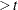are given a missing value in X1 and are subsequently removed from the risk set. Computationally, this approach is not as efficient as the one that uses the counting process formulation.